Physical Chemistry : Viscosity and Flow Mechanics

Example Questions

Example Question #1 : Phases And Properties Of Matter

An object is floating on water. Which of the following is true?

I. The object has a lower density than water

II. The buoyancy force would be higher if the object sank

III. The volume of fluid displaced would be higher if the object sank

II only

II and III

I and III

I and II

I and III

Explanation:

Recall than an object floats on water if it has a lower density than water. A floating or submerged object in a liquid has a gravitational pull (downward force) equal to the buoyancy force (upward force). The force due to gravity (gravitational pull) depends only on the mass of the object. In this case, the object can never be submerged because the density of the solid cannot be changed (unless the phase of the object is changed). To submerge, the object has to be placed in a liquid with LOWER density.

The volume of fluid displaced depends on the volume occupied by the object in the liquid. When an object floats, only part of the object is inside the liquid. This means that only part of the object’s volume is occupied inside the liquid (lower volume of fluid displaced). If the object were to be submerged (in a different liquid), the entire volume of the object would be inside the liquid and there would be an increase in the volume of fluid displaced.

Example Question #1 : Phases And Properties Of Matter

Fluid A and Fluid B flow through the same pipe. However, it is found that fluid A flows much slower than fluid B. What can you conclude about fluid A and fluid B?

None of these

Both of these

The forces between layers in Fluid A is lower

Fluid A has a higher coefficient of viscosity

Fluid A has a higher coefficient of viscosity

Explanation:

Viscosity is a measure of resistance to flow. A liquid with high viscosity is said to have strong forces between its layers, making it harder to flow whereas a liquid with low viscosity has weak forces between its layers, making it easier to flow. Viscosity is quantified by the coefficient of viscosity (higher the coefficient, higher the viscosity and higher the resistance to flow). The question states that fluid A has more trouble flowing; therefore, fluid A has higher coefficient of viscosity and stronger forces between layers.

Example Question #2 : Phases And Properties Of Matter

According to Poiseuille’s principle, the flow rate __________ as viscosity increases and the flow rate __________ as length of pipe increases.

decreases . . . decreases

increases . . . increases

decreases . . . increases

increases . . . decreases

decreases . . . decreases

Explanation:

Poiseuille’s principle quantifies the flow rate of a fluid through a pipe to numerous variables as follows.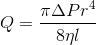where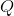is the flow rate,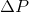is change in pressure,is radius,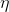is viscosity, andis length. We can see that viscosity and length terms are in the denominator; therefore, flow rate and these two terms are inversely related. This means that increasing viscosity and/or length of pipe will decrease the flow rate (and vice versa). Note that the radius has the biggest exponential factor; therefore, altering radius of the pipe will contribute to the biggest change in flow rate.

Example Question #1 : Phases And Properties Of Matter

One end of a pipe (End A) has twice the radius as the second end (End B). What can you conclude about the flow of fluid through this pipe?

Fluid will flow faster and have a higher pressure at End B

Fluid will flow faster and have a lower pressure at End A

Fluid will flow faster and have a higher pressure at End A

Fluid will flow faster and have a lower pressure at End B

Fluid will flow faster and have a lower pressure at End B

Explanation:

To solve this question, we need to use the continuity equation and Bernoulli’s equation. The continuity equation is as follows: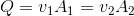whereis flow rate,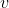is velocity, andis the area. This equation states that that the flow rate of a fluid is the same, regardless of changes in velocity and area. The equation implies that as the area is increased (for example,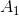) the velocity (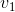) decreases to maintain a constant flow rate and vice versa. The question states that End A has the larger radius; therefore, the velocity is lower at End A.

Bernoulli’s equation is as follows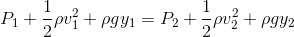whereis pressure,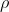is density,is velocity,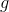is acceleration due to gravity, andis the height from ground. This equation implies that as velocity is increased on one side, the pressure decreases to compensate. Since we already determined that End B has the higher velocity, it will also have the lower pressure.

All Physical Chemistry Resources